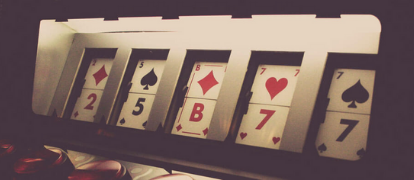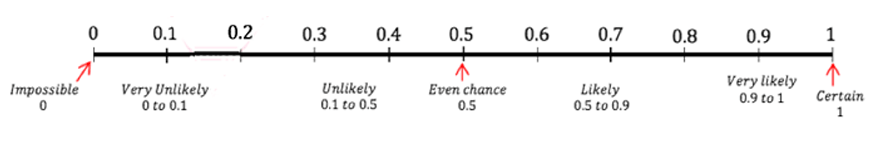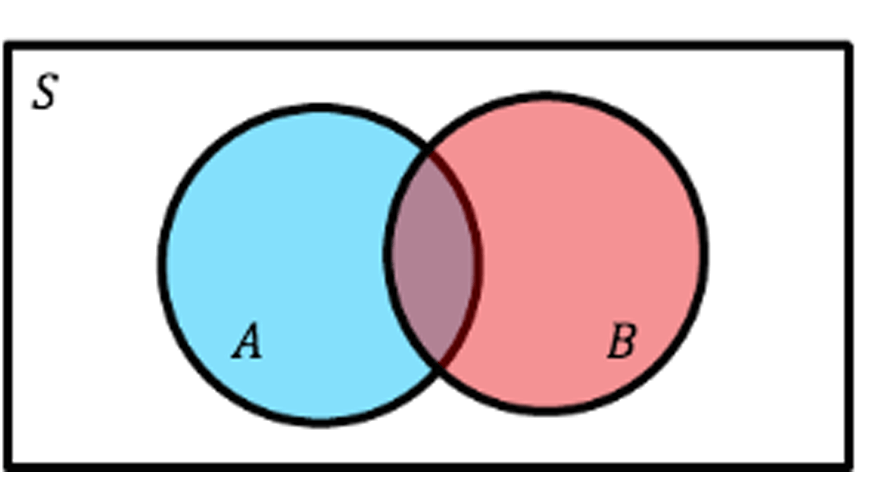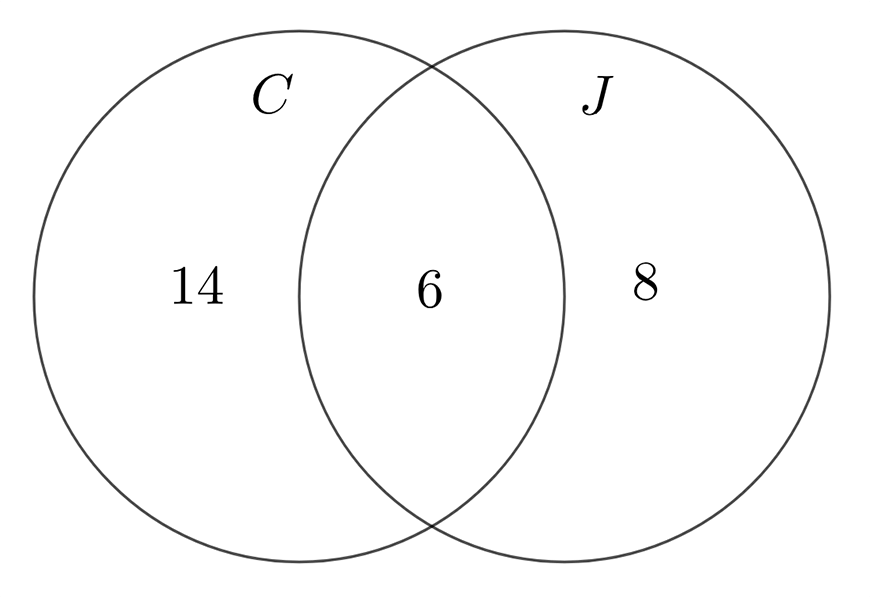# Part 2: Year 10 Probability

In this article, we give you the rundown on probability so you can increase your odds of acing Year 10 Maths!## Year 10 Probability

In this article, we walk you through Year 10 probability and explain what it is and how to tackle the various problems you will encounter. This article will give you a comprehensive overview of how probability works and how to work out the odds of certain events occurring.

At the end of this article, we’ve got some concept check questions for you to test what you’ve learned. After you’ve answered them you can check your answers.

## Syllabus Outcomes

• Selects appropriate notations and conventions to communicate mathematical ideas and solutions MA5.2-1WM
• Interprets mathematical or real-life situations, systematically applying appropriate strategies to solve problems MA5.2-2WM
• Constructs arguments to prove and justify results MA5.2-3WM
• Describes and calculates probabilities in multi-step chance experiments MA5.2-17SP

What does this mean for you? Simply that in this unit, you will calculate probabilities in real-life and hypothetical situations.

• Introduction to probability
• Probability with compound events
• Using Venn diagrams in probability

## Assumed Knowledge

No prior knowledge on probability is assumed

## Year 10 Probability

Probability is a number between 0 and 1 assigned to an event. This number indicates how likely this event is to occur. What these numbers mean is indicated in the probability spectrum below.If we want to say the probability of tossing a heads is $$50%$$, we write that $$P(heads)=0.5$$.

## Sample Space

The sample space is a list of all possible outcomes of a certain scenario.

Here is sample space for rolling a dice.

$$\text{Sample Space} \ 1: \{1,2,3,4,5,6\}$$

There are many ways you can write out the sample space as a list of outcomes.

All of them are perfectly valid if they list out all the possibilities.

$$\text{Sample Space} \ 2: \{(1 \ \text{or } 4 \ \text{or} \ 5),(2 \text{ or} \ 3),6\}$$ (only 3 outcomes)

$$\text{Sample Space} \ 3:\{\text{evens, odds}\}$$

## Want to revise Maths but short on time?

Don’t fear! With our 2-Day Maths Holiday Course for Year 10 students, you will consolidate your existing knowledge and equip yourself with new Maths skills to ace your next exam!## Events and Outcomes

An event is a list of outcomes in a sample space.

For example, $$\{1,2\}$$ is an event for $$\text{Sample Space 1}, \{(2 \ \text{or } 3),5\}$$ is an event for $$\text{Sample Space 2}$$ and $$\{\text{evens, odds}\}$$ is an event for $$\text{Sample Space 3}$$.

An event can even have zero outcomes, one outcome or be the entire sample space.

## Finding the Probability of an Event

$$P(\text{Event})=\frac{\text{Total Outcomes in Event}}{\text{Total Outcomes in Sample Space}}$$  (*)

*This formula only works if every outcome in the sample space is equally likely.

$$\text{Sample Space 2}$$ will never work as the outcomes $$(2 \text{ or } 3)$$ and $$5$$ are not equally likely.

Let’s use this formula to find out the probability that we roll a $$3$$ or a $$6$$.

We must pick a sample space we can use.

$$\text{Sample Space 3}$$ doesn’t work as we can’t write our event as a list of outcomes.

$$\text{Sample Space 1}$$ works.

Let’s list our information.

• $$\text{Event}={3,6}$$
• $$\text{Total Outcomes in Event}=2$$
• $$\text{Total Outcomes in Sample Space}=6$$

∴$$P(\text{Event})=\frac{2}{6}=\frac{1}{3}$$

This method works for every single “simple” probability question.

Here is a summary of the steps.

1. Write the event as a list of outcomes
2. Write the sample space as a list of outcomes
3. Apply the formula

### Examples

1. What is the probability of rolling a $$1, 2, 3$$ or $$4$$?

(Answer=$$2 \over 3$$)

2. What is the probability of flipping a tails?

(Answer$$= \frac{1}{2}$$)

3. What is the probability of rolling an even number?

a) Using Sample Space 1

b) Using Sample Space 3

c) Which method was faster?

(Answer=$$1 \over 2$$)

## Complementary Events

Every event $$E$$ has a complementary event $$\bar{E}$$.

$$\bar{E}$$ is the event that $$E$$ does not occur.

### Examples:

$$E=\text{it will rain} ↔ \bar{E}=\text{it will not rain}$$ $$E= \text{rolling a 1,2,3 or 4} ↔ \bar{E}= \text{rolling a 5 or 6}$$

The probability of a complimentary event is given by the formula

$$P(\bar{E} )=1-P(E)$$

### Questions

1. Given that the probability of winning a soccer game is $$0.7$$, what is the probability of losing the soccer game?

(Answer=$$0.3$$)

2. We know from before that the probability of rolling a $$1, 2, 3$$ or $$4$$ is $$2 \over 3$$. Hence what is the probability of rolling a $$5$$ or $$6$$?

(Answer=$$\frac{1}{3}$$)

## Compound Events

Most Year 10 probability questions can be solved using the following two formulae.

Formula 1: $$P(A \text{ and } B)=P(A)\times P(B) (*)$$

Formula 2: $$P(A \text{ or } B)=P(A)+P(B)-P(A \text{ and } B)$$

* Only works if $$A$$ and $$B$$ are independent of each other. To understand these formulae we need to understand two concepts.

## Dependent and Independent Events

An event $$B$$ is dependent on $$A$$ if the $$P(B)$$ depends on whether or not A occurs.

### Examples:

1. Let $$A$$ be the event that it rains on Monday, and let $$B$$ be the event that it rains on Tuesday.

If it rains on Monday, it is more likely that it will rain on Tuesday.

Therefore $$P(B)$$ will change depending if $$A$$ happens or not.

Thus $$B$$ is dependent on $$A$$.

2. I have a deck of cards. Let $$A$$ be the event I draw a red card on the first draw.

Let $$B$$ be the event I draw a red card on the second draw. (After my first draw, I don’t replace the card).

If $$A$$ happens, there are less red cards than black cards before my second draw.

Therefore $$P(B)$$ is lower.

If $$A$$ doesn’t happen (i.e. I draw a black card on the first go), there are more red cards than black cards.

Therefore $$P(B)$$ is higher.

Hence, $$P(B)$$ will change depending on if $$A$$ happens or not.

Thus $$B$$ is dependent on $$A$$. An event $$B$$ can only be dependent on $$A$$ if it happens after $$A$$ in time.

Events $$A$$ and $$B$$ are independent of each other if $$A$$ is not dependent on $$B$$ and $$B$$ is not dependent on $$A$$.

### Question:

1. I flip a coin twice in a row.

Let $$A$$ be the event I flip heads on the first flip. Let $$B$$ be the event I flip heads on the second flip.

Are $$A$$ and $$B$$ independent?

Is $$A$$ dependent on $$B$$?

The outcome of one coin flip doesn’t affect the outcome of the other coin flip.

Therefore $$A$$ and $$B$$ are independent.

## OR and AND

If we have two events $$A$$ and $$B$$, we can create two new events called $$A$$ or $$B$$ and $$A$$ and $$B$$.

### Examples:

1. Let $$A$$ be the event that it rains on Monday and $$B$$ be the event that it rains on Tuesday.

Therefore $$A$$ or $$B$$ is the event that it rains at all on either Monday or Tuesday.

$$A$$ and $$B$$ is the event that it rains on both Monday and Tuesday.

2. Let $$A$$ be the event of rolling a $$1, 2, 3$$ or $$4$$. Let $$B$$ be the event of rolling a $$4$$ or $$5$$.

Therefore $$A$$ or $$B$$ is rolling a $$1, 2, 3, 4$$ or $$5$$ and $$A$$ and $$B$$ is rolling a $$4$$.

If $$P(A \ \text{and} \ B)=0$$, then $$A$$ and $$B$$ are mutually exclusive.

This means that $$A$$ and $$B$$ can’t both occur.

## Solving Problems

### Question 1:

You roll two dice at once. What is the probability of rolling a double $$6$$?

What the question is asking is find $$P(\text{first die}=6 \ \text{and second die}=6)$$. As the outcomes of the dice don’t depend on each other, they are independent. Therefore we can use Formula 1.

 $$P(\text{first die}=6 \ \text{and second die}=6)=P(\text{first die}=6) \times P(\text{second die}=6)$$

Now we just need to find $$P(\text{first die}=6)$$ and $$P(\text{second die}=6)$$. Using the methods in the previous section, we can evaluate these to be both $$\frac{1}{6}$$.

Thus we can find our answer;

 $$P(\text{first die}=6 \ \text{and second die}=6)=(\frac{1}{6}) \times (\frac{1}{6})$$ $$=\frac{1}{36}$$

Therefore the probability of rolling a double $$6$$ is $$\frac{1}{36}$$.

### Question 2:

You roll two dice at once. What is the probability of rolling at least one $$6$$?

What the question is asking is find $$P(\text{first die}=6 \text{ or second die}=6)$$.

As we know $$P(\text{first die}=6 \ \text{and second die}=6)$$ we can use Formula 2.

 $$P(\text{first die}=6 \ \text{or second die}=6) \\ =P(\text{first die}=6)+P(\text{second die}=6) – P(\text{first die}=6 \ \text{and second die}=6) \\ =\frac{1}{6}+\frac{1}{6}-\frac{1}{36}=\frac{11}{36} \\$$

Concept Check Questions

1. What is the probability I flip two heads in a row?

(Answer=$$1 \over 4$$)

2. I play two tennis matches. The probability I win any tennis match is $$0.4$$.

2a. What is the probability I win the first match and lose the second match?

(Answer=$$0.24$$)

2b. What is the probability I win at least one match?
(Hint. Find $$P(\text{win match 1 or win match 2})$$)

(Answer=$$0.64$$)

3. I roll two dice. What is the probability I roll at least one even number?

(Answer=$$3 \over 4$$)

## Using Complementary Events

Questions with “at least” can be easily solved using complementary events.

Look out for questions that ask for the probability of “at least” and “none”. These will be complements of each other.

For example, the complement of “having at least one sibling” is “having no siblings”, and the complement of “selecting no girls” is “selecting at least one girl”.

In other words, “at least one” is the complement of “nothing”.

If you are given questions that tell you the probability of getting “at least one”, you will know that the probability of getting nothing is $$1-P(\text{at least one})$$

### Question:

The probability of obtaining at least one head when a number of coins are tossed is $$0.99$$ What is the probability of obtaining no heads?

Since the probability of “at least one” is $$0.99$$, the probability of obtaining nothing will be the complement:

$$1-P(\text{at least one head}) = 1-0.99 = 0.01$$

## Probability with Venn Diagrams

Venn diagrams are a helpful visual tool for helping solve probability questions involving non-mutually exclusive events (that is, both events can occur at the same time).The rectangle is the sample space $$S$$.

All numbers inside the rectangle must add to the number of outcomes in the sample space

### Question:

1. In a group of $$35$$ students, $$20$$ study Chinese, $$14$$ study Japanese and $$7$$ do not study either languages.

Represent this information in a Venn diagram.

What is the probability that a student chosen at random will study:

a) Both languages?

b) Chinese or Japanese?Often, the question will not tell you how many outcomes are in the overlapping region in the middle.

We will have to solve this ourselves.

We know that the numbers inside the rectangle have to add up to $$35$$, as there are only $$35$$ outcomes. $$7$$ students definitely go outside the two circles (events), as they study neither language.

How do we know how to fill out the remaining $$3$$ parts?

An easy way to solve it is to add the $$3$$ numbers up: $$20 + 14 + 7 = 41$$.

This exceeds the number of outcomes by $$6$$ – thus, we know that the number inside the overlap will be $$6$$.

Now that we know $$6$$ students study both Chinese and Japanese, we can deduce that $$14$$ students study ONLY Chinese and $$8$$ students study ONLY Japanese.

We can fill out the rest of the table!

As a quick check in the end, we can also add our numbers up:

 $$7 \ (\text{neither}) + 14 \ (\text{Chinese only}) + 8 \ (\text{Japanese only}) + 6 \ (\text{both}) = 35$$

This diagram can then be used to answer the follow-up questions:

1.

 $$P(\text{both languages})=\frac{\text{Number of students studying both languages}}{\text{Total number of students}}\\ =\frac{6}{35}$$

2.

 $$P(\text{Chinese or Japanese})=\frac{\text{No.of students studying Chinese ONLY}+\text{No.of students studying Japanese ONLY}}{\text{Total number of students}}\\ =\frac{22}{35}$$

## Need to consolidate your trigonometry?

In our next article, we discuss trigonometric ratios.

© Matrix Education and www.matrix.edu.au, 2021. Unauthorised use and/or duplication of this material without express and written permission from this site’s author and/or owner is strictly prohibited. Excerpts and links may be used, provided that full and clear credit is given to Matrix Education and www.matrix.edu.au with appropriate and specific direction to the original content.